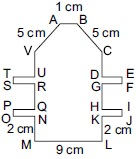# User Forum

Subject :IMO    Class : Class 5

In the given figure, VU = RQ = CD = GH = 2.5 cm, DE = GF = HI = KJ = ON = PQ = SR = TU = 3 cm and EF = IJ = TS = PO = 0.5 cm.
Find the perimeter of the given figure (not drawn to scale).A60 cm
B70 cm
C80 cm
D50 cm